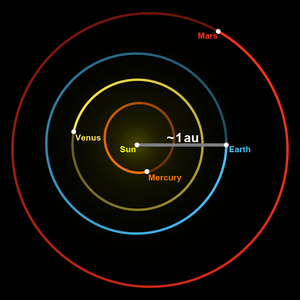# Astronomical unit facts for kids

Kids Encyclopedia Facts
Quick facts for kids
Astronomical unitThe grey line indicates the Earth–Sun distance, which on average is about 1 astronomical unit.
General information
Unit system Astronomical system of units
(Accepted for use with the SI)
Unit of length
Symbol au or AU
Conversions
1 au or AU in ... ... is equal to ...
metric (SI) units    1.495978707×1011 m
imperial & US units    9.2956×107 mi
astronomical units    4.8481×10−6 pc
1.5813×10−5 ly

The astronomical unit (AU) is a unit of length derived from the Earth's orbit. It is the average distance the Earth gets from the Sun on the long axis of the ellipse.

Its definition is: the length of the semi-major axis of the Earth's elliptical orbit around the Sun. "Semi-major" means half the long axis.

The AU is about 150 million kilometers or 93 million miles. Astronomers usually measure distances within the Solar System in astronomical units. Mars is about 1.4 AU from the Sun, Jupiter lies at roughly 5.2 AU, and Neptune is roughly 30 AU from the Sun. Light travels an AU in about 8.317 minutes.

## Images for kidsIn Spanish: Unidad astronómica para niños